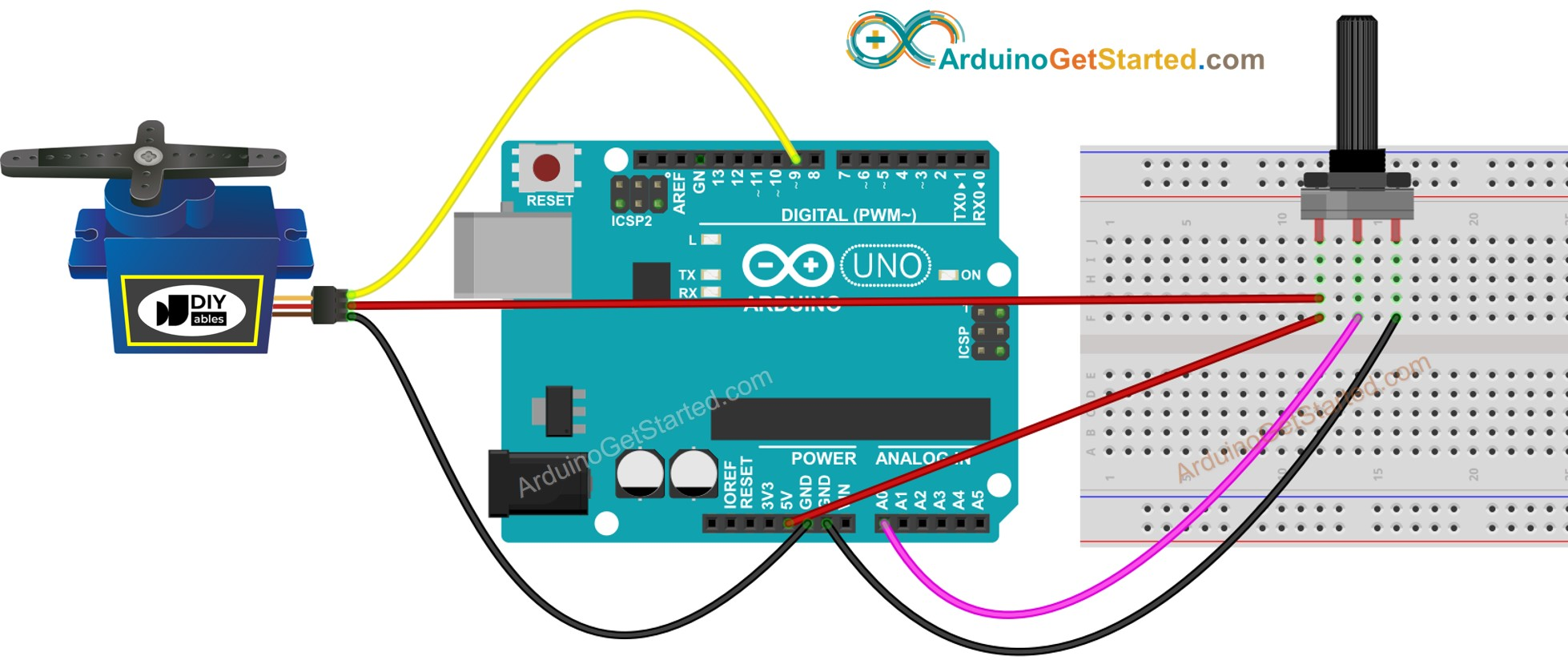# Arduino - Potentiometer Triggers Servo Motor

In a previous tutorial, We have learned how to rotate servo motor according to the potentiometer's output value. In this tutorial, We are going to learn how to:

• If the potentiometer's analog value is greater than a threshold, rotate servo motor to 90 degree
• If the potentiometer's analog value is lower than a threshold, rotate servo motor back to 0 degree.
• If the potentiometer's output voltage is greater than a threshold, rotate servo motor to 90 degree
• If the potentiometer's output voltage is lower than a threshold, rotate servo motor back to 0 degree.

## Hardware Required

Please note: These are affiliate links. If you buy the components through these links, We may get a commission at no extra cost to you. We appreciate it.

## About Servo Motor and Potentiometer

If you do not know about servo motor and potentiometer (pinout, how it works, how to program ...), learn about them in the following tutorials:

## Wiring DiagramThis image is created using Fritzing. Click to enlarge image

## Arduino Code - Analog Threshold

/* * Created by ArduinoGetStarted.com * * This example code is in the public domain * * Tutorial page: https://arduinogetstarted.com/tutorials/arduino-potentiometer-triggers-servo-motor */ #include <Servo.h> // constants won't change const int POTENTIOMETER_PIN = A0; // Arduino pin connected to Potentiometer pin const int SERVO_PIN = 9; // Arduino pin connected to Servo Motor's pin const int ANALOG_THRESHOLD = 500; Servo servo; // create servo object to control a servo void setup() { servo.attach(SERVO_PIN); // attaches the servo on pin 9 to the servo object servo.write(0); } void loop() { int analogValue = analogRead(POTENTIOMETER_PIN); // read the input on analog pin if(analogValue > ANALOG_THRESHOLD) servo.write(90); // rotate servo motor to 90 degree else servo.write(0); // rotate servo motor to 0 degree }

### Quick Steps

• Connect Arduino to PC via USB cable
• Open Arduino IDE, select the right board and port
• Copy the above code and open with Arduino IDE
• Click Upload button on Arduino IDE to upload code to Arduino
• Rotate the potentiometer
• See the change of servo motor

## Arduino Code - Voltage Threshold

Potentiometer's analog value is converted to voltage value, and then compared to voltage threshold to triggers servo motor

/* * Created by ArduinoGetStarted.com * * This example code is in the public domain * * Tutorial page: https://arduinogetstarted.com/tutorials/arduino-potentiometer-triggers-servo-motor */ #include <Servo.h> // constants won't change const int POTENTIOMETER_PIN = A0; // Arduino pin connected to Potentiometer pin const int SERVO_PIN = 9; // Arduino pin connected to Servo Motor's pin const float VOLTAGE_THRESHOLD = 2.5; // Voltages Servo servo; // create servo object to control a servo void setup() { servo.attach(SERVO_PIN); // attaches the servo on pin 9 to the servo object servo.write(0); } void loop() { int analogValue = analogRead(POTENTIOMETER_PIN); // read the input on analog pin float voltage = floatMap(analogValue, 0, 1023, 0, 5); // Rescale to potentiometer's voltage if(voltage > VOLTAGE_THRESHOLD) servo.write(90); // rotate servo motor to 90 degree else servo.write(0); // rotate servo motor to 0 degree } float floatMap(float x, float in_min, float in_max, float out_min, float out_max) { return (x - in_min) * (out_max - out_min) / (in_max - in_min) + out_min; }

## Video Tutorial

We are considering to make the video tutorials. If you think the video tutorials are essential, please subscribe to our YouTube channel to give us motivation for making the videos.

※ OUR MESSAGES